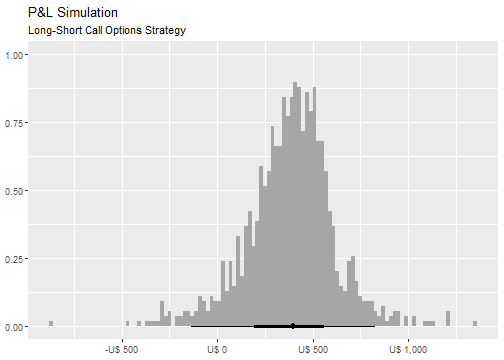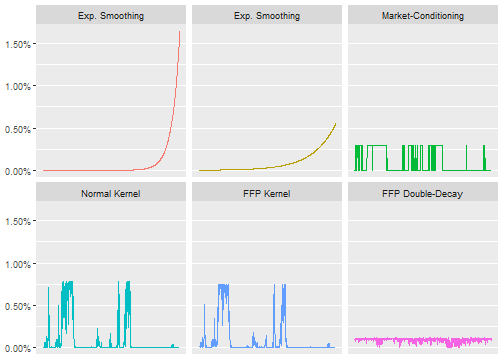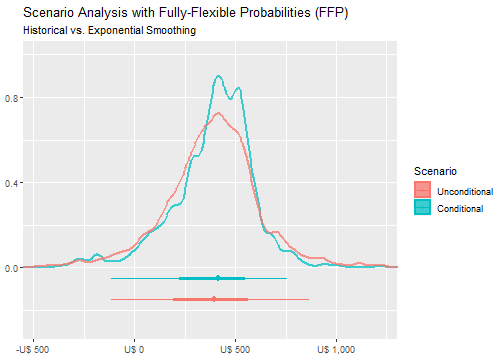Consider, as in Historical Scenarios with Fully Flexible Probabilities, that we would like to price and stress-test a book of long-short call options, in which the underlying is the S&P500 index. The db_tbl dataset contains information on the interest-rates, US stock market and implied volatility. It also provides $$6$$ macroeconomic variables that can be used to filter the state of the economy.

### Prepare the Data

# Load Packages
library("dplyr")  # Data Manipulation and pipe (%>%)
library("ffp")    # Fully-Flexible Probabilities
data("db_tbl")
db_tbl
#> # A tibble: 1,083 x 9
#>    SWAP2YR SWAP10YR CDXIG OTR S&P 500 Dollar Index Crude  Gold   VIX
#>      <dbl>    <dbl>       <dbl>     <dbl>          <dbl> <dbl> <dbl> <dbl>
#>  1    4.13     4.48        52.4     1218.           86.3  67.6  445.  13.6
#>  2    4.18     4.52        52.6     1233.           86.6  66.0  446.  12.9
#>  3    4.24     4.58        50.8     1236.           87.0  64.4  446   12.5
#>  4    4.26     4.58        51.1     1232.           87.0  64.5  448.  12.9
#>  5    4.25     4.55        50.5     1241.           86.9  64.1  450   12.0
#>  6    4.29     4.60        49.8     1241.           87.7  63.3  451.  11.6
#>  7    4.24     4.56        49.7     1231.           87.8  63.1  447   12.4
#>  8    4.25     4.59        49.5     1227.           87.7  65.1  451.  12.9
#>  9    4.28     4.64        49.3     1228.           88.1  64.8  456.  12.5
#> 10    4.37     4.72        50       1238.           88.1  63    460.  11.2
#> # ... with 1,073 more rows, and 1 more variable: 10YR Inflation Swap Rate <dbl>


The variables VIX, SWAP10YR and S&P 500 are essential inputs in the Black-Scholes formula and offer an interesting starting point for scenario generation. By making them “stationary” and feeding the output into the last available observation, we get a distribution that “mimmics” historical randomness.

# Inputs in Black-Scholes option pricing
invariants <- db_tbl %>%
dplyr::select(VIX, SWAP10YR, S&P 500) %>%
purrr::map_df(~diff(log(.))) # compute the continuous return for every column

# get last observations
last_obs <- db_tbl %>%
dplyr::slice_tail(n = 1) %>%
dplyr::select(VIX, SWAP10YR, S&P 500)

# Last observation for the underlying (SP500)
S_0   <- last_obs[["S&P 500"]]
# Last observation for the implied-volatility
vol_0 <- last_obs[["VIX"]]
# Last observation for the risk-free rate
rf_0  <- last_obs[["SWAP10YR"]]

# Gererate Paths from Historical Scenarios
paths <- purrr::map2_df(.x = last_obs, .y = invariants, .f = ~ .x * exp(.y)) %>%
dplyr::rename(S_T = S&P 500, vol_T = VIX, rf_T = SWAP10YR) %>%
tibble::rowid_to_column(var = "id")
paths
#> # A tibble: 1,082 x 4
#>       id vol_T  rf_T   S_T
#>    <int> <dbl> <dbl> <dbl>
#>  1     1  16.3  3.85 1188.
#>  2     2  16.6  3.86 1176.
#>  3     3  17.7  3.82 1169.
#>  4     4  15.9  3.79 1183.
#>  5     5  16.7  3.86 1172.
#>  6     6  18.2  3.78 1164.
#>  7     7  17.8  3.84 1169.
#>  8     8  16.6  3.86 1174.
#>  9     9  15.4  3.88 1183.
#> 10    10  18.5  3.79 1167.
#> # ... with 1,072 more rows


The 10-years inflation swap-rate is also extracted from the data to be used as a macro-conditioning variable.

inflation <- db_tbl %>%
dplyr::select(10YR Inflation Swap Rate) %>%
dplyr::slice(1:(nrow(db_tbl) - 1))


The call-price function can be constructed as follows:

call_price <- function(p, k, r, t, s) {
d_1 <- log(p / k) + (r + s * s / 2) * t
d_2 <- d_1 - s * sqrt(t)
c <- p * stats::pnorm(d_1) - k * exp(-r * t) * stats::pnorm(d_2)
c
}


In which the arguments match the standard in the literature: $$p$$ is for price, $$k$$ for strike, $$r$$ for the risk-free rate, $$t$$ for time and $$s$$ for the volatility.

It' assumed that every option has $$21$$ days to expiry and the market offers grid of $$20$$ different strikes homogeneously distributed from deeply-in to slightly out-of-the money.

N <- 20
# call parameters
K      <- S_0 * (seq(0.8, 1.1, length = N))
Expiry <- (2:(N + 1)) / 252


The trading opportunities are assessed on a daily basis: at the beginning of the trading day a grid of 10 different in-the-money options are bought and a grid of 10 different out-of the money options are sold (object u).

# Market Pricing
pnl <- tibble::tibble(K = K, Expiry = Expiry, panel = as.factor(1:20), S_0, vol_0, rf_0) %>%
dplyr::mutate(paths = list(paths)) %>%
tidyr::unnest(paths) %>%
dplyr::select(id, panel, dplyr::everything()) %>%
# portfolio scenarios
dplyr::mutate(
# Pricing
call_open  = call_price(p = S_0, k = K, r = rf_0, t = Expiry, s = vol_0),
call_close = call_price(p = S_T, k = K, r = rf_T, t = Expiry - 1 / 252, s = vol_T),
pnl        = call_close - call_open,
# Units to buy and sell
u          = rep(c(1, -1), each = nrow(paths) * 10)
) %>%
# Aggregate by "day" (here represented by the "id" variable)
dplyr::group_by(id) %>%
dplyr::summarise(pnl_u = as.double(pnl %*% u))
pnl
#> # A tibble: 1,082 x 2
#>       id pnl_u
#>    <int> <dbl>
#>  1     1 533.
#>  2     2 482.
#>  3     3 256.
#>  4     4 687.
#>  5     5 473.
#>  6     6 169.
#>  7     7 210.
#>  8     8 491.
#>  9     9 773.
#> 10    10  99.1
#> # ... with 1,072 more rows


The histogram shows the marginal distribution of this strategy when every scenario has the same probability of occurrence, which is the standard approach:

pnl %>%
ggplot2::ggplot(ggplot2::aes(x = pnl_u)) +
ggdist::stat_histinterval(breaks = 100, outline_bars = TRUE) +
ggplot2::scale_x_continuous(labels = scales::dollar_format(prefix = "U$")) + ggplot2::labs(title = "P&L Simulation", subtitle = "Long-Short Call Options Strategy", x = NULL, y = NULL)### Flexible Probabilities Now, Fully-Flexible Probabilities (FFP) kicks in: #### Full Information #### # exponential-smoothing fp_es1 <- exp_decay(invariants, 0.0166) fp_es2 <- exp_decay(invariants, 0.0055) # crisp-conditioning on inflation fp_cc <- crisp(inflation, lgl = as.logical(inflation >= 2.8)) # normal kernel on inflation fp_kd <- kernel_normal(inflation, mean = 3, sigma = var(diff(inflation[]))) #### Partial Information #### # entropy-pooling by kernel-dumping on inflation fp_ekd <- kernel_entropy(inflation, mean = 3, sigma = var(diff(inflation[]))) # entropy-pooling by moment-matching fp_emc <- double_decay(invariants, slow = 0.0055, fast = 0.0166)  Six different objects are estimated with varying degrees of flexibility, as the image shows:### Comparison and Results The new marginal distribution of the P&L that results from “tweaking” probabilities can be visualized, case by case, with scenario_density(). scenario_density(pnl$pnl_u, fp_es2) +
ggplot2::scale_x_continuous(labels = scales::dollar_format(prefix = "U\$ ")) +
ggplot2::labs(title = "Scenario Analysis with Fully-Flexible Probabilities (FFP)",
subtitle = "Historical vs. Exponential Smoothing")As the figure shows, a slightly superior average return and a smaller interquartile range arises from exponentiating probabilities with half-life of 6 months.

Finally, the main statistics of the scenarios at hand can be assessed with empirical_stats().

empirical_stats(pnl %>% dplyr::select(pnl_u), fp_es1)
#> # A tibble: 6 x 3
#>   stat  name   value
#>   <fct> <chr>  <dbl>
#> 1 Mu    pnl_u 394.
#> 2 Std   pnl_u 183.
#> 3 Skew  pnl_u  -1.28
#> 4 Kurt  pnl_u   5.62
#> 5 VaR   pnl_u 259.
#> 6 CVaR  pnl_u 297.